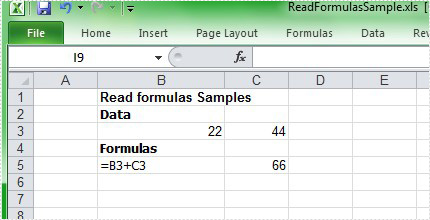Read Excel Formulas in C#, VB.NET

Introduction

After we wrote formulas for Excel, the values would be displayed in specified cells. It is possible that the formulas are wrongly used or lost because there are too many formulas. Therefore, sometimes, we need to read the formula for one cell to ensure if the formula is right. Well then, how to read Excel formula?

Through Formulas tab in Excel 2007, we can find one button named Show Formulas. Select the cells which we want to read formulas, and then click the button. After that, we can see the formulas instead of values.

Besides using the button, we can also use keyboard shortcut to read formulas in Excel. Select the cells where we want to show the formulas, and then press Ctrl + ` to read.

Spire.XLS presents you an easy way to read formula in the worksheet. You can get the formula through the value you provide, while you should specify where the value is. In the demo, we load a workbook from file named "ReadFormulaSample.xls" which has a formula written in the sheet["B5"], we can read the formula through its value in sheet["C5"]. In this example, in order to view, we read the formula to the sheet["D5"].

The following code displays the method to read formulas for cells with C#/VB.NET:

[C#]
using Spire.Xls;

{
class Program
{
static void Main(string[] args)
{
//Create a new workbook
Workbook workbook = new Workbook();

//Initialize the worksheet
Worksheet sheet = workbook.Worksheets;

sheet.Range["D5"].Text = sheet.Range["C5"].Formula;

//Save the file
workbook.SaveToFile("sample.xls",ExcelVersion.Version97to2003);

//Launch the file
System.Diagnostics.Process.Start("Sample.xls");
}
}
[VB.NET]
Imports Spire.Xls

Module Module1

Sub Main()
'Create a new workbook
Dim workbook As New Workbook()

'Initialize the worksheet
Dim sheet As Worksheet = workbook.Worksheets(0)

sheet.Range("D5").Text = sheet.Range("C5").Formula

'Save doc file.
workbook.SaveToFile("Sample.xls",ExcelVersion.Version97to2003)

'Launching the MS Word file.
System.Diagnostics.Process.Start("Sample.xls")
End Sub
End Module

After running the demo, you may find a formula appear in the worksheet you specify: